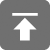# 工学1号馆

home

## Project Euler 46–Goldbach’s other conjecture

Wu Yudong    August 20, 2018     欧拉计划   494

Goldbach’s other conjecture

It was proposed by Christian Goldbach that every odd composite number can be written as the sum of a prime and twice a square.

9 = 7 + 2×12
15 = 7 + 2×22
21 = 3 + 2×32
25 = 7 + 2×32
27 = 19 + 2×22
33 = 31 + 2×12

It turns out that the conjecture was false.

What is the smallest odd composite that cannot be written as the sum of a prime and twice a square?

9 = 7 + 2×12
15 = 7 + 2×22
21 = 3 + 2×32
25 = 7 + 2×32
27 = 19 + 2×22
33 = 31 + 2×12

//（Problem 46）Goldbach's other conjecture
// Completed on Fri, 26 Jul 2013, 16:58
// Language: C11
//
// 版权所有（C）wu yudong
// 博客地址：http://www.wuyudong.com
#include<stdio.h>
#include<math.h>
#include<string.h>
#include<ctype.h>
#include<stdlib.h>
#include<stdbool.h>

bool issquare(int n)  //判断一个自然数是否为一个平方数
{
if (ceil(sqrt(n)) * ceil(sqrt(n)) == n)
return true;
else
return false;
}

bool isprim(int n)				//素数判断
{
for (int i = 2; i * i <= n; i++) {
if (n % i == 0)
return false;
}
return true;
}

bool judge(long long n)
{
int i = 1;
long long t;
while ((t = (n - 2 * (i * i))) > 0) {
if (isprim(t))
return true;
i++;
}
return false;
}

int main()
{
for (long long i = 1001; i < 100000000; i = i + 2) {
if (!isprim(i) && !judge(i)) {
printf("%lld\n", i);
break;
}
}
return 0;
}